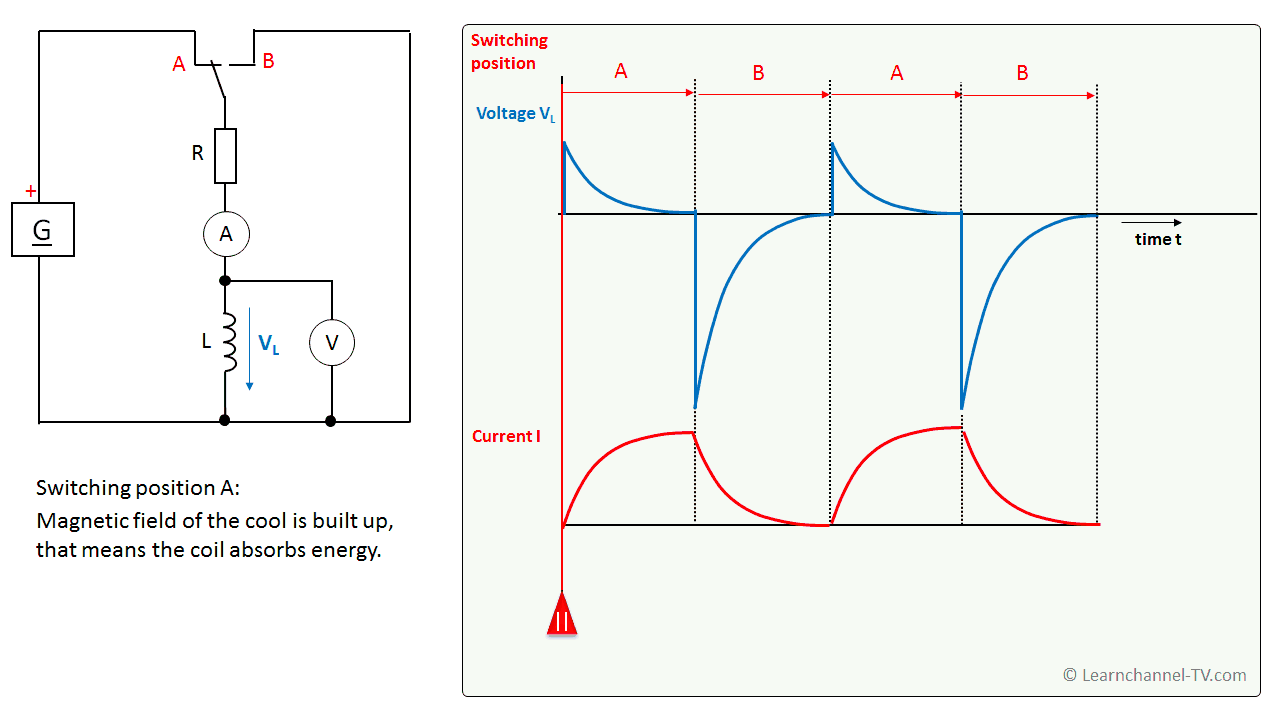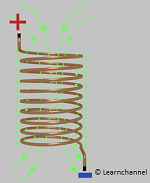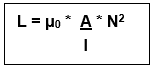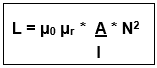# Inductors in DC Circuits

## Inductors in DC Circuits

Test: The DC voltage at the coil is switched on and off. The voltage curve at the coil and the current are measured.Inductor in a DC Circuits

If the voltage at the coil is switched off, this means a large change in current. The coil reacts to this and induces a voltage, the so-called self-induction voltage, which counteracts the change in current. In other words: The coil tries to maintain the current flow.

A coil has an inductance L of 1 H (pronounced "Henry") when a self-induction voltage of 1 V is generated in one second with a constant change in current of 1 A.

Formula symbol Inductance: L Unit: Henry H or Ωs

The self-inductance voltage is proportional to the change in current over time. It is calculated by:

Vind = -    = - L dI
.              dt           dt

Note: The term inductance is also generally used as an umbrella term for the coil.

Caution when switching off inductors! The self-induction voltage can be several times higher than the supply voltage!

### Factors Affecting InductanceMagnetic field of a coil

The coil can store energy in the form of a magnetic field. The ability of the coil to build up this magnetic field, i.e. inductance, depends on the current strength and the geometric properties of the coil itself.

Following factors affect the inductance of a coil:

• Number of wire turns in the coil - the greater the number of turns of wire in the coil, the greater amount of magnetic field force and therefore the greater the inductance.
• Coil Area - greater coil area means less opposition to the formation of magnetic field flux for a given amount of field force
• Coil Length - the shorter the coil’s length, the greater the inductance. (considering the straight coil)

The formula for calculating an inductance is as follow (considering an Air Core Coil):Where:

L = inductance
µ0 = Permeability of Free Space µ0 = 4π 10−7 (H/m) or 1,257 * 10-6 Vs/Am
N = number of turns on the coil
A = the cross-sectional area of the core in square meters
l = length of the coil in meters

If we insert a core of a ferromagnetic material the inductive properties of the coil will increase. The factor by which the magnetic conductivity increases is described by the so-called relative permeability μr. So, our formula changes to:Considering the energy of a coil:

The greater the inductance L of a coil, the higher the energy it can store in its magnetic field. The equation for this is:

E = 1/2  L  *  I2

Example: An air coil has the following characteristics: Number of turns 10000, length 20 cm, cross-sectional area 20 cm2. What is the inductance of the coil?